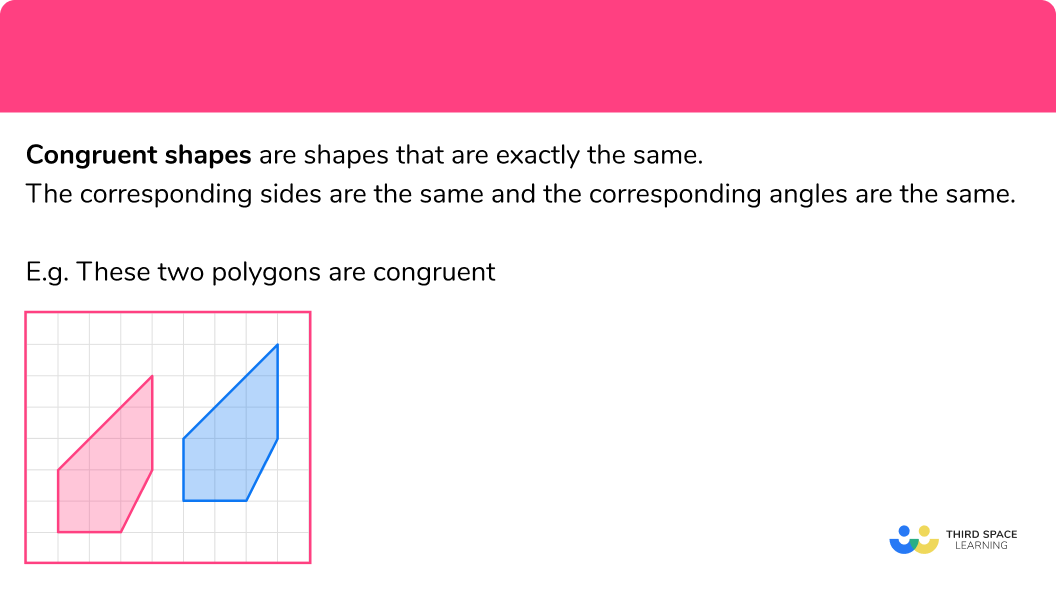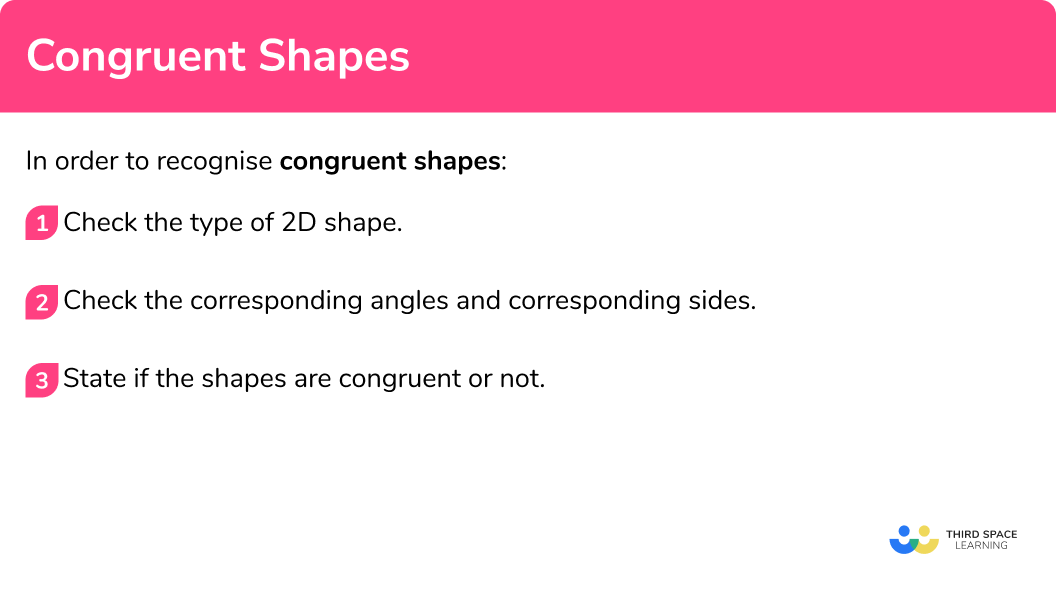# Congruent Shapes

Here we will learn about congruent shapes, including what they are and how to recognise them.

There are also congruent shapes worksheets based on Edexcel, AQA and OCR exam questions, along with further guidance on where to go next if you’re still stuck.

## What are congruent shapes?

Congruent shapes are shapes that are exactly the same.

The corresponding sides are the same and the corresponding angles are the same.

To do this we need to check all the angles and all the sides of the shapes. If two shapes are congruent they will  fit exactly on top of one another.

E.g.

These two polygons are congruent.

The red shape has been translated to give the blue shape.

E.g.

The red shape has been reflected to give the blue shape.

E.g.

These two polygons are congruent.

The red shape has been rotated to give the blue shape.

E.g.

These two polygons are NOT congruent. They are similar.

The red shape has been enlarged by multiplying by a scale factor to give the blue shape.

If two shapes are the same but different sizes, one being an enlargement of the other, these are known as similar shapes.

### What are congruent shapes?### Congruent triangles

There are four conditions to be able to prove if a pair of triangles are congruent.

Reasons for congruency:

SSS (three sides the same),

RHS (right-angled triangle, hypotenuse and a side the same),

ASA or AAS (two angles and one side the same),

SAS (side-angle-side, two sides and the included angle the same).

E.g.

These two triangles are congruent triangles.

They have two angles that are the same.

The side in between the angles is also equal.

The congruence condition would be angle-side-angle (which is abbreviated to ASA).

## How to recognise congruent shapes

In order to recognise congruent shapes:

1. Check the type of 2D shape.
2. Check the corresponding angles and corresponding sides.
3. State if the shapes are congruent or not.

### Explain how to recognise congruent shapes### Related lessons on congruence and similarity

Congruent shapes is part of our series of lessons to support revision on congruence and similarity. You may find it helpful to start with the main congruence and similarity lesson for a summary of what to expect, or use the step by step guides below for further detail on individual topics. Other lessons in this series include:

## Congruent shapes examples

### Example 1: congruent shapes

Are these 2D shapes congruent?

1. Check the type of 2D shape.

Both shapes are rectangles.

2Check the corresponding angles and corresponding sides.

All the angles are 90^{\circ} .

The short sides on both rectangles are 1.

The long sides on both rectangles are 3.

3State if the shapes are congruent or not.

The shapes are the shape shape and the same size – they are congruent shapes.

### Example 2: congruent shapes

Are these 2D shapes congruent?

Check the type of 2D shape.

Check the corresponding angles and corresponding sides.

State if the shapes are congruent or not.

### Example 3: congruent shapes

Are these 2D shapes congruent?

Check the type of 2D shape.

Check the corresponding angles and corresponding sides.

State if the shapes are congruent or not.

### Example 4: congruent shapes

Are these 2D shapes congruent?

Check the type of 2D shape.

Check the corresponding angles and corresponding sides.

State if the shapes are congruent or not.

### Example 5: congruent shapes

Are these 2D shapes congruent?

Check the type of 2D shape.

Check the corresponding angles and corresponding sides.

State if the shapes are congruent or not.

### Example 6: congruent shapes

Are these 2D shapes congruent?

Check the type of 2D shape.

Check the corresponding angles and corresponding sides.

State if the shapes are congruent or not.

### Common misconceptions

• Shapes can be congruent but in different orientations

The second shape may be in a different orientation to the first shape. The shapes can still be congruent. Perhaps use tracing paper to help you check.

• Shapes can be congruent but mirror images

The second shape may be a mirror image of the first shape. The shapes can still be congruent. Perhaps use tracing paper to help you check.

Some shapes on a grid can be tricky. Use the straight lines on the grid to help you identify right angles and work out the side lengths. Be careful with the diagonals.

• In most diagrams the diagrams are NOT drawn to scale

Questions about congruent shapes are often on grids. But sometimes diagrams may have shapes which are NOT drawn to scale. So use the measurements given, rather than measuring for yourself.

### Practice congruent shapes questions

1. Which shape is congruent to shape X?ABCDThe original shape is a rectangle with sides 1 and 4 . So has shape C.

2. Which shape is congruent to shape X?ABCDShape A is the same as the original shape, but has been rotated.

3. Which shape is congruent to shape X?ABCDThe original shape is a rectangle with sides 3 and 4. So has shape D.

4. Which shape is congruent to shape X?ABCDShape B is the same as the original shape, but is upside down.

5. Which shape is congruent to shape X?ABCDShape C is the same as the original shape, but is a reflection.

6. Which shape is congruent to shape X?ABCDShape C is the same as the original shape.

### Congruent shapes GCSE questions

1. Which shape is congruent to shape X?(1 mark)

Shape A

(1)

2. Which shape is congruent to shape A?(1 mark)

Shape Y

(1)

3. Which shape is congruent to shape M?(1 mark)

Shape D

(1)

## Learning checklist

You have now learned how to:

• What congruent shapes are
•  How to identify congruent shapes

## Still stuck?

Prepare your KS4 students for maths GCSEs success with Third Space Learning. Weekly online one to one GCSE maths revision lessons delivered by expert maths tutors.

Find out more about our GCSE maths tuition programme.